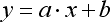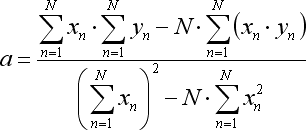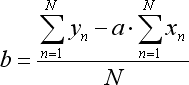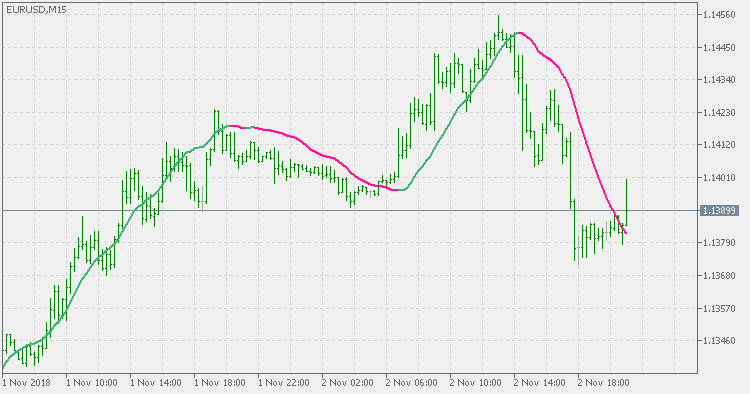Interesting script?
So post a link to it -
let others appraise it

You liked the script? Try it in the MetaTrader 5 terminal# Liner regression - indicator for MetaTrader 5

Views:
4076
Rating:
Published:
2018.11.02 20:14
Updated:
2019.01.29 16:01

Theory:

Linear regression attempts to model the relationship between two variables by fitting a linear equation to observed data. One variable is considered to be an explanatory variable, and the other is considered to be a dependent variable. For example, a modeler might want to relate the weights of individuals to their heights using a linear regression model. The equation is the following:Where x is a number of bars and y are prices.

The ratios of the mentioned equation are calculated as follows:There are quite a few good implementations (some are explained in a very good way here : 3 Methods of Indicators Acceleration by the Example of the Linear Regression but there are some issues that needed to be addressed (mainly that some methods are not suitable for usage on in-code data ...).

This version:

This is "yet another" method that is producing exact results (without an approximation) as the "to the last letter" method, that can be used on arbitrary data and is fast (it is faster than even the method that is using built in functions to speed up the calculation). Execution time does not depend on the calculation period (it is negligible slower for very large periods than the calculation for short periods) which makes it suitable for usage in all sorts of filtering / calculating / smoothing ...

Usage:

You can use the color change as a signalVolume Profil & Range V6

Volume Profile + Range v6.0 (former TPO).CCI variation

CCI variationWeis Waves

Volume Wave indicator originally idealized by Richard D. Wyckoff.Corrected generalized DEMA

Corrected generalized DEMA